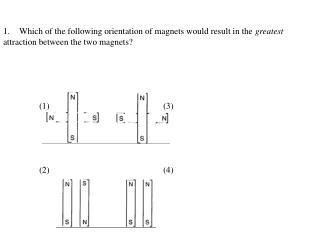# 2. The magnetic field of a magnet: (1) affects all objects. (2) is visible. - PowerPoint PPT PresentationDownload Presentation2. The magnetic field of a magnet: (1) affects all objects. (2) is visible.

2. The magnetic field of a magnet: (1) affects all objects. (2) is visible.
Download Presentation## 2. The magnetic field of a magnet: (1) affects all objects. (2) is visible.

- - - - - - - - - - - - - - - - - - - - - - - - - - - E N D - - - - - - - - - - - - - - - - - - - - - - - - - - -
##### Presentation Transcript

1. 1. Which of the following orientation of magnets would result in the greatest attraction between the two magnets? (1) (3) (2) (4)

2. 2. The magnetic field of a magnet: (1) affects all objects. (2) is visible. (3) only affects magnetic materials. (4) would have no effect on a compass placed near the magnet. 3. The law of conservation of charge means that: (1) new charge can be made easily. (2) new charge can be made through friction. (3) charge can be destroyed. (4) charge can neither be created nor destroyed but simply moved around.

3. 4. Like magnetic poles (1) attract. (2) repel. (3) are a North and a South pole. (4) are found in the middle of a magnet. 5. Which of the following best explains why socks just removed from a dryer often cling together? (1) The socks have like poles. (2) The socks have unlike poles. (3) The socks have like charges. (4) The socks have unlike charges.

4. 6. An electric current is able to produce (1) mass (2) inertia (3) gravity (4) magnetism 7. Magnetism is most closely related to (1) heat (2) inertia (3) gravity (4) electricity

5. 8. What is the power source in the drawing of the circuit below?   (1) bulb (2) conductor (3) switch (4) battery

6. 9. Electricity is the flow of tiny particles called: (1) Protons (2) Neutrons (3) Electrons (4) Atoms 10. In the diagram below, if bulb 3 is removed then bulb 4 will (1) stay lit because it is a series circuit. (2) go out because it is a parallel circuit. (3) stay lit because it is a parallel circuit. (4) go out because it is a series circuit.

7. 11. Which diagram would result in a working circuit once the switch is closed?

8. 12. A conductor (1) leads a band or orchestra. (2) is always made of metal. (3) allows charge to flow through it easily. (4) prevents charge from flowing through it.

9. 13. The type of circuit which has only one path for electrons to flow is known as a (1) singular circuit. (2) direct circuit. (3) series circuit. (4) parallel circuit.

10. 14. A direct electrical current is different from an alternating current because only the direct electrical current (1) produces a high voltage. (2) flows without resistance. (3) allows the flow of electrons in one direction. (4) can be converted to other forms of energy.

11. 15. Which of the following circuits will light the bulb?

12. 16. In this diagram if bulb 1 goes out, bulb 2 will (1) go out because there is only one path for the current to flow. (2) go out because there are several paths for the current to flow. (3) stay lit because it is a series circuit. (4) stay lit because there are several paths for the current to flow.

13. 17. In the drawing, light bulbs X and Y are wired in parallel and connected to battery B so that both bulbs are lit. Which statement best describes what would happen if light bulb X burned out? (1) The battery would no longer be usable. (2) The socket holding light bulb X would become warmer. (3) Light bulb Y would also burn out. (4) Light bulb Y would continue to be lit.

14. 18. Which is the best way to generate an electrical current in a coil of wire? (1) stroking the coil with a piece of silk (2) heating the coil uniformly (3) laying the coil near a magnet (4) rotating the coil in a magnetic field

15. 19. In the diagram below, what could be true about the electrical charges on A and B? (1) A is positive and B is negative. (2) A is positive and B is positive. (3) A is negative and B is positive. (4) Both A and B are neutrally charged.

16. 20. The picture shows how to make an electromagnet. Which of these would make the electromagnet strongest? (1) Using a longer wire from the battery to the nail. (2) Wrapping the wire around the nail more times. (3) Wrapping the wire around a nonmetallic object. (4) Making the loops of wire less tightly coiled.

17. 21. Jamie does an experiment. She charges a pencil by rubbing it with a piece of silk. Jamie finds that she can use the pencil to attract the silk. Why does the attraction occur?

18. 22. In the diagram below, use the concept of electrical charges to explain what is happening with the balloons in the diagram below.

19. 24. Describe a circuit.